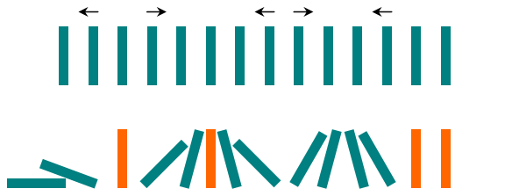There are `N` dominoes in a line, and we place each domino vertically upright.

In the beginning, we simultaneously push some of the dominoes either to the left or to the right.After each second, each domino that is falling to the left pushes the adjacent domino on the left.

Similarly, the dominoes falling to the right push their adjacent dominoes standing on the right.

When a vertical domino has dominoes falling on it from both sides, it stays still due to the balance of the forces.

For the purposes of this question, we will consider that a falling domino expends no additional force to a falling or already fallen domino.

Given a string “S” representing the initial state. `S[i] = 'L'`, if the i-th domino has been pushed to the left; `S[i] = 'R'`, if the i-th domino has been pushed to the right; `S[i] = '.'`, if the `i`-th domino has not been pushed.

Return a string representing the final state.

Example 1:

Example 2:

Note:

1. `0 <= N <= 10^5`
2. String `dominoes` contains only `'L`', `'R'` and `'.'`

• 如果遇到`L`，那么就把之前的`.`设置成`L`
• 如果遇到`R`，那么就往后搜索`L`
• 如果没有`L`了，那么就把后面的全部设置成`R`
• 如果遇到了`R`，那么这两个`R`之间的`.`就全部设置成`R`，把新的`R`当成新的起点
• 如果遇到了`L`，那么就从两边的`R``L`向中间合拢，直到相遇